• 主要介绍了Python编程实现线性回归批量梯度下降法代码实例，具有一定借鉴价值,需要的朋友可以参考下
• # 批量梯度下降 def gradDescent ( dataMatIn , classLabels ) : """ :param dataMatIn: 是一个2维Numpy数组，每行代表每个训练样本，每列分别代表样本不同特征 :param classLabels: 是一个List，是训练...
一、逻辑回归代码
逻辑回归其实是求一个分类器，是二分类问题，其利用一个sigmoid函数去定义样本属于正类的概率，sigmoid函数的输入值z利用了线性回归的wx，所得出的数值是在[0,1]内的概率，即样本属于正类的概率值。在实践中，我们需要从训练样本中学习出一个w和b，这就是需要的逻辑回归模型，w和b包含在一个权重系数向量w中，w的更新是依据对损失函数即负对数似然函数求偏导数得梯度，然后每轮迭代中w根据负梯度来更新，梯度是一个向量，表示函数值上升最快的方向，由于这里的损失函数（负对数似然函数）是凸函数，因此在训练中求使其值最小的w值，就利用到梯度下降法（负梯度方向），当迭代到一定次数后所得到的w即为模型，预测时将利用sigmoid函数（此时输入值即wx，w是所得模型，x是预测样本）求得x属于正样本的概率（回归），若值大于阈值，即判定为正类（分类）。
关于回归预测模型和损失函数的定义，以及梯度更新公式的推导，
参考：https://mp.weixin.qq.com/s/BJxdDz7DQg5QIWzzgNQrgA
其梯度公式最后少了一个负号
其中每轮迭代中权重系数矩阵weights的更新运用到训练样本数据矩阵dataMatrix，训练样本标签矩阵labelMat和训练样本预测值矩阵h，这种向量化或矩阵化运算提高计算效率，避免for循环带来的计算开销，其矩阵计算的原理如下：这里样本特征是2，加上偏置项1，所以有3个特征，故其权重向量的维度也是3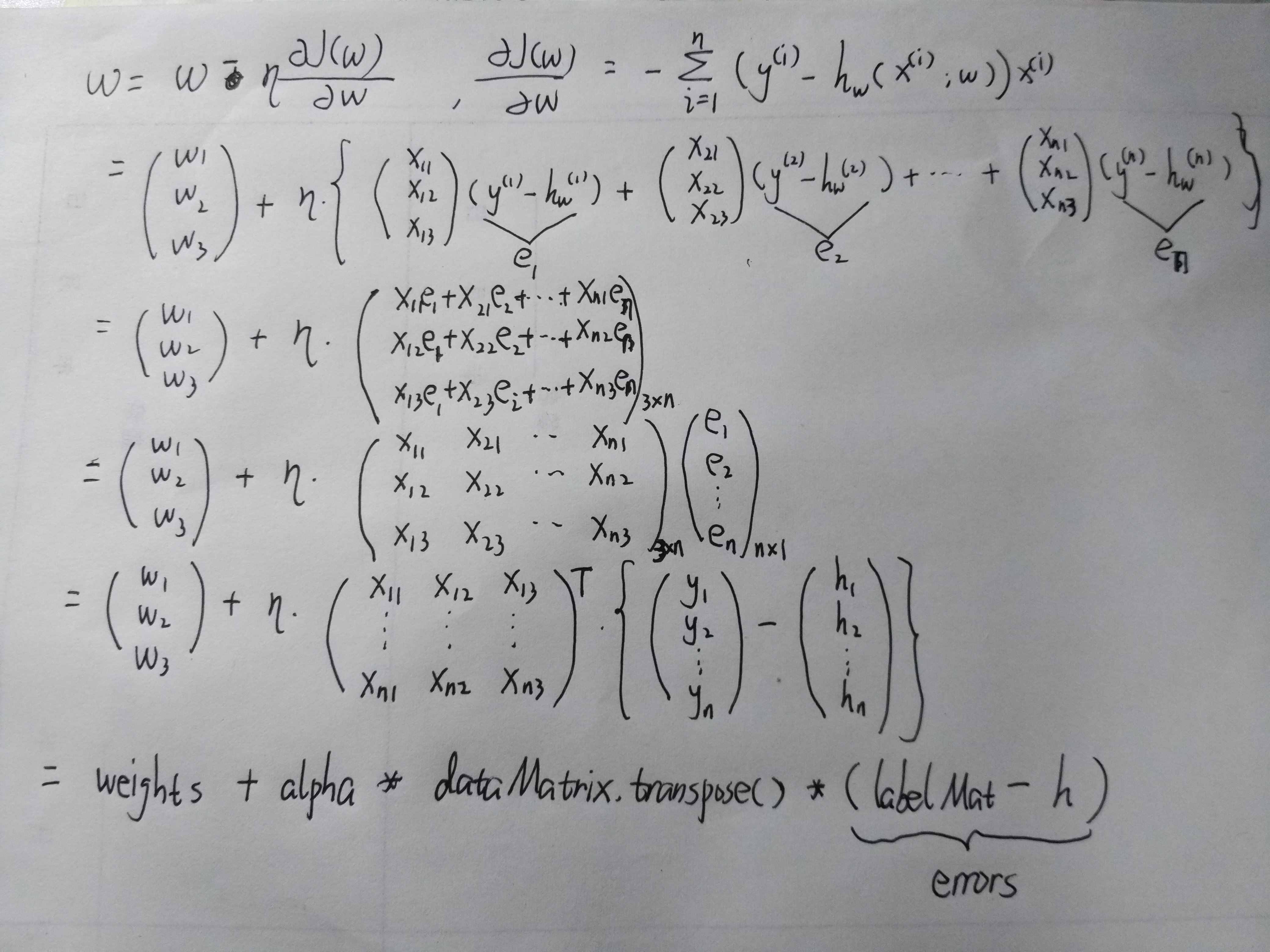完整的逻辑回归代码如下：
# -*- coding: utf-8 -*-
import numpy as np
import matplotlib.pyplot as plt
def loadDataSet(file_name):
"""
:desc: 加载并解析数据
:param file_name: 文件名称，要解析的文件所在路径
:return:
dataMat -- 原始数据的特征
labelMat -- 原始数据的标签，也就是每条样本对应的类别
"""
dataMat = []
labelMat = []
fr = open(file_name)
for line in fr.readlines():
lineArr = line.strip().split()
if len(lineArr) == 1:
continue
dataMat.append([1.0, float(lineArr), float(lineArr)])  # 此处为每个样本添加一个特征1.0
labelMat.append(int(lineArr))
return dataMat, labelMat

# sigmoid跃阶函数
def sigmoid(x):

return 1.0 / (1 + np.exp(-x))

# 批量梯度下降
def gradDescent(dataMatIn, classLabels):
"""
:param dataMatIn: 是一个2维Numpy数组，每行代表每个训练样本，每列分别代表样本不同特征
:param classLabels: 是一个List，是训练样本的类别标签，它是一个1*100的行向量。为了便于矩阵计算，需要将该行向量转换为列向量，
即将原向量转置后赋值给labelMat
:return: Numpy数组，权重系数
"""
# 将numpy数组dataMatIn转化为numpy矩阵[[1 1 2]
#                                   [1 1 2]
#                                    ...  ]
dataMatrix = np.mat(dataMatIn) # 转换为Nupmy矩阵
# 将标签List转化为行矩阵[[0 1 0 1 0 1 ...]]，并转置成列矩阵[
#                                                
#                                                
#                                                ..]
labelMat = np.mat(classLabels).transpose()
# m是训练样本个数，n是特征数
m, n = np.shape(dataMatrix)
# alpha代表移动步长
alpha = 0.001
# 迭代次数
maxCycles = 500
# 初始权重系数矩阵,长度与特征数相同[[1.]
#                              [1.]
#                              [1.]]
weights = np.ones((n, 1))  # numpy数组
for k in range(maxCycles):
h = sigmoid(dataMatrix*weights)  # 对应于训练样本的预测值 100*1的列矩阵
errors = labelMat - h # 100*1的列矩阵
weights = weights + alpha * dataMatrix.transpose() * errors
return np.array(weights)

# 随机梯度下降
# 梯度下降算法在每次更新权重系数时都需要遍历整个数据集，计算复杂度较高
# 随机梯度下降每次更新权重只需要一个样本
def stocGradDescent(dataMatrix, classLabels):
"""

:param dataMatrix: Numpy2维数组
:param classLabels: List
:return: numpy数组，权重系数
"""
m, n = np.shape(dataMatrix) # m是第一维度的元素个数，即样本数，n是第二维度元素个数，即特征数
dataMat = np.mat(dataMatrix)
alpha = 0.001
weights = np.ones((n, 1))
print(np.shape(weights))
print(np.shape(dataMat))
for i in range(m):
h = sigmoid(np.sum(dataMat[i]*weights))  # 取一条样本与权重系数相乘，这里的h是一个数值而不再是矩阵了
error = classLabels[i] - h
weights = weights + alpha * error * dataMat[i].transpose()
return np.array(weights)

# 改进随机梯度下降
def stocGradDescent1(dataMatrix, classLabels, numIter=150):
"""
:param dataMatrix: Numpy2维数组
:param classLabels: List
:param numIter: 迭代次数
:return:
"""
m, n = np.shape(dataMatrix)
dataMat = np.mat(dataMatrix)
weights = np.ones((n, 1))
for j in range(numIter):
dataIndex = list(range(m)) # 存放样本索引[0 1 2 .. m-1]
for i in range(m):
alpha = 4/(1.0+j+i)+0.0001  # alpha 会随着迭代不断减小
# 随机取一个样本的索引,其值在[0，len(dataIndex))左闭右开区间范围内
randIndex = int(np.random.uniform(0, len(dataIndex)))
h = sigmoid(np.sum(dataMat[dataIndex[randIndex]]*weights))
error = classLabels[dataIndex[randIndex]] - h
weights = weights + alpha * error * dataMat[dataIndex[randIndex]].transpose()
# 删除该随机索引
del dataIndex[randIndex]
return np.array(weights)

def plotBestFit(dataArr, labelMat, weights):
"""
Desc: 将训练样本和训练出的权重系数可视化
:param dataArr: numpy2维数组，样本数据的特征，[[1 1 2] [1 2 1] [1 1 2]]
:param labelMat: List，样本数据的类别标签
:param weights: numpy数组，权重系数
:return: None
"""
n = np.shape(dataArr)  # 样本个数
xcord1 = []; ycord1 = []  # 分别存放类别为1的样本特征
xcord2 = []; ycord2 = []  # 分别存放类别为2的样本特征
for i in range(n):
if int(labelMat[i]) == 1:
xcord1.append(dataArr[i, 1]); ycord1.append(dataArr[i, 2])
else:
xcord2.append(dataArr[i, 1]); ycord2.append(dataArr[i, 2])
fig = plt.figure()
ax = fig.add_subplot(111)
ax.scatter(xcord1, ycord1, s=30, c='red', marker='s')
ax.scatter(xcord2, ycord2, s=30, c='green')
x = np.arange(-3.0, 3.0, 0.1)
y = (-weights - weights * x) / weights
ax.plot(x, y)
plt.xlabel('X')
plt.ylabel('Y')
plt.savefig("D://fig_2.png")
plt.show()

def testLR():
#  1.准备训练数据
dataMat, labelMat = loadDataSet("D:\\testSet.TXT")
#  2.训练模型
dataArr = np.array(dataMat)
# weights = gradDescent(dataArr, labelMat)
weights = stocGradDescent1(dataArr, labelMat)

print("The final weight is: \n", weights, "\n", type(weights), np.shape(weights))
# 数据可视化
plotBestFit(dataArr, labelMat, weights)

#  预测样本
def classifyVector(x, weights):
"""

:param x: numpy matrix, 待预测的样本特征向量
:param weights: numpy array, 根据训练样本学习由梯度下降得到的权重
:return: 如果prob大于0.5返回1，否则返回0
"""
prob = sigmoid(np.sum(x*weights))
if prob > 0.5:
return 1.0
else:
return 0.0

if __name__ == "__main__":
testLR()

其中用于学习权重系数有三种方法：分别是批量梯度下降gradDescent、随机梯度下降stocGradDescent和改进随机梯度下降stocGradDescent1。批量梯度下降也就是常说的梯度下降法，梯度下降算法在每次更新回归系数时都需要遍历整个数据集，该方法在处理 100 个左右的数据集时尚可，但如果有数十亿样本和成千上万的特征，那么该方法的计算复杂度就太高了。一种改进方法是一次仅用一个样本点来更新回归系数，该方法称为 随机梯度下降算法。相比之下，批量梯度下降比随机梯度下降具有更好的学习效果，学习得到的回归系数用作预测的效果更准确，下面是两种算法寻优后的分类超平面：
由于w0*x0+w1*x1+w2*x2=f(x)， x0最开始就设置为1， x2就是我们画图的y值，令f(x)=0，
所以： w0+w1*x+w2*y=0 => y = (-w0-w1*x)/w2 即分类超平面直线

批量梯度下降：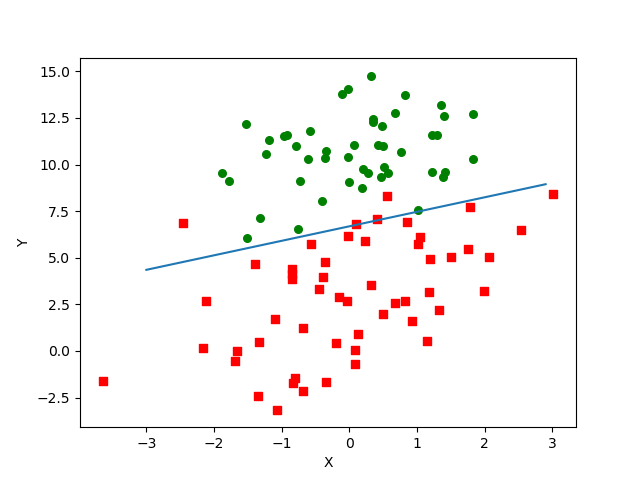随机梯度下降：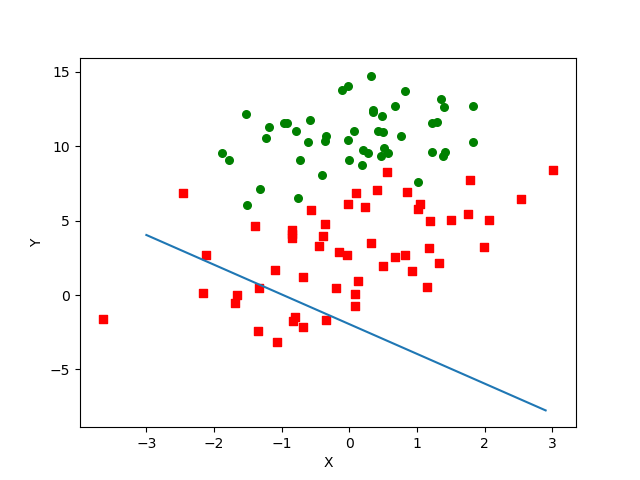随机梯度下降每次迭代只用到一条样本数据，其下降方向并不是全局最优的，也就是不一定向着最低点的方向，因此虽然迭代速度快，但是很容易陷入局部最优，最终的学习效果就没有批量梯度下降好。
因此对随机梯度下降作一次改进，代码里是stocGradDescent1，第一处改进为 alpha 的值。alpha 在每次迭代的时候都会调整，会随着迭代次数不断减少，但永远不会减小到 0，因为在计算公式中添加了一个常数项。
第二处修改为 randIndex 更新，这里通过随机选取样本来更新回归系数。这种方法每次随机从列表中选出一个值，然后从列表中删掉该值（再进行下一次迭代）。。
改进随机梯度下降：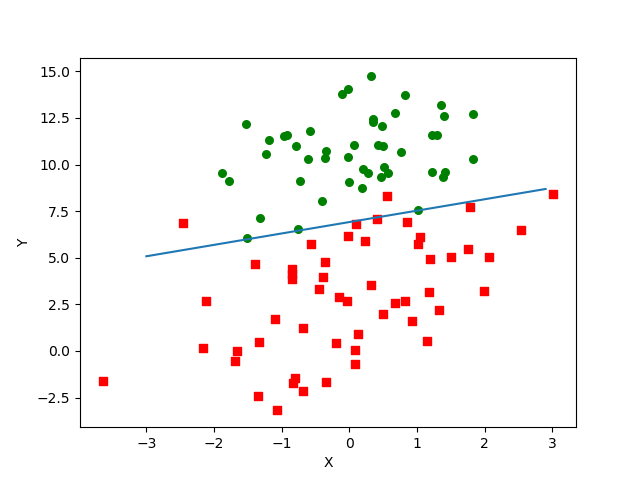在机器学习领域，梯度下降分为3类：
1、批量梯度下降BGD：每轮迭代计算所有样本数据，所有样本共同决定最优下降方向
优点：逼近全局最优
缺点：计算量大，批量完成，速度慢，样本数量大时不适用
2、随机梯度下降SGD：每轮迭代从训练样本中抽取一个样本进行计算更新，每次都不用遍历所有数据集
优点：速度快，逐条样本进行计算
缺点：陷入局部最优，下降幅度相对慢，需要迭代更多次数，因为每次选取的方向不一定是最优
3、小批量的梯度下降MBGD：每轮迭代计算所有数据中的子集，是1和2的中和方案，节省时间，并且寻优也准确
二、从疝气病症预测病马的死亡率
使用逻辑回归来预测患有疝病的马的存活问题。疝病是描述马胃肠痛的术语。然而，这种病不一定源自马的胃肠问题，其他问题也可能引发马疝病。这个数据集中包含了医院检测马疝病的一些指标，分为训练集和测试集，每条数据 共22个字段，最后一个字段是其类别标签0或1，项目代码如下
病马数据集：
链接：https://pan.baidu.com/s/19qLbQ2TL757r0zyJjbLTUQ
提取码：gw3j
#  预测样本
def classifyVector(x, weights):
"""

:param x: numpy matrix, 待预测的样本特征向量
:param weights: numpy array, 根据训练样本学习由梯度下降得到的权重
:return: 如果prob大于0.5返回1，否则返回0
"""
prob = sigmoid(np.sum(x*weights))
if prob > 0.5:
return 1.0
else:
return 0.0

#  从疝气病症预测病马的死亡率
def colicTest():
"""
Desc：读入测试集和训练集，并对数据进行格式化处理
:return: errorRate 分类错误率
"""
frTrain = open('D:\\horseColicTraining.txt')  # 训练样本
frTest = open('D:\\horseColicTest.txt')  # 测试样本
trainingSet = [] # [[],[],[],...]
trainingLabels = []
for line in frTrain.readlines():
currLine = line.strip().split('\t') # 得到每一条数据字符串列表（共22个字段，最后一个字段为标签）
lineArr = [] # 存储每条样本特征
for i in range(len(currLine)-1):
lineArr.append(float(currLine[i]))
trainingSet.append(lineArr)
trainingLabels.append(float(currLine[len(currLine)-1]))
# 使用改进后的随机梯度下降算法求最佳权重系数
# trainWeights = stocGradDescent1(np.array(trainingSet), trainingLabels, 500) # 改进后随机梯度下降
# trainWeights = stocGradDescent(np.array(trainingSet), trainingLabels) # 随机梯度下降
trainWeights = gradDescent(np.array(trainingSet), trainingLabels) # 梯度下降
errorCount = 0
numTestVec = 0.0

# 读取测试样本进行测试，计算分类错误的样本条数和最终的错误率
for line in frTest.readlines():
numTestVec += 1.0 # 测试样本数量加1
currLine = line.strip().split('\t')
lineArr = [] # 存储每条样本特征
for i in range(len(currLine)-1):
lineArr.append(float(currLine[i]))
if int(classifyVector(np.mat(lineArr), trainWeights)) != int(currLine[len(currLine)-1]):
errorCount += 1 # 预测错误，错误数加1

errorRate = float(errorCount) / numTestVec
print("测试的错误率是: ", errorRate)
return errorRate
if __name__ == "__main__":
colicTest()

运行结果：
批量梯度下降的错误率：0.29850746268656714
随机梯度下降的错误率：0.47761194029850745
改进随机梯度的错误率：0.3283582089552239


展开全文• arr)), regr.loss_arr, marker='o', c='green') plt.show() 一元线性回归 越往下写的越好 # _*_ coding: utf-8 _*_ ''' 一元线性回归Python实现 ''' import numpy as np import pandas as pd import matplotlib....
 一元线性回归

# _*_ coding : utf-8 _*_
import numpy as np
import pandas as pd
import matplotlib.pyplot as plt

class LinearRegression(object):
def __init__(self,learning_rate=0.01,max_iter=100,seed=None):
np.random.seed(seed)
self.lr = learning_rate
self.max_iter = max_iter
self.w = np.random.normal(1,0.1)
self.b = np.random.normal(1,0.1)
self.loss_arr = []
def fit(self,x,y):
self.x = x
self.y = y
for i in range(self.max_iter):
self._train_step()
self.loss_arr.append(self.loss())
def model(self,x,w,b):
return x * w + b
def predict(self,x=None):
if x is None:
x = self.x
y_pred = self.model(x,self.w,self.b)
return y_pred
def loss(self,y_true=None,y_pred=None):
if y_true is None or y_pred is None:
y_true = self.y
y_pred = self.predict(self.x)
return np.mean((y_true - y_pred)**2)
def _calc_gradient(self):
d_w = np.mean((self.x * self.w + self.b - self.y) * self.x)
d_b = np.mean((self.x * self.w + self.b - self.y))
return d_w,d_b
def _train_step(self):
d_w,d_b = self._calc_gradient()
self.w = self.w - self.lr * d_w
self.b = self.b - self.lr * d_b
return self.w,self.b

def generate_data():
np.random.seed(272)
data_size = 100
X = np.random.uniform(low=1.0,high=10.0,size=data_size)
y = X * 20 + 10 + np.random.normal(loc=0.0,scale=10.0,size=data_size)
return pd.DataFrame({"X":X,"y":y})

if __name__ == '__main__':
data = np.array(generate_data())
x = data[:,0]
y = data[:,1]
regr = LinearRegression(learning_rate=0.01,max_iter=10,seed=111)
regr.fit(x,y)

def show_data(x, y, w=None, b=None):
plt.scatter(x, y, marker='.')
if w is not None and b is not None:
plt.plot(x, w * x + b, c='red')
plt.show()

show_data(x, y, regr.w, regr.b)
plt.scatter(np.arange(len(regr.loss_arr)), regr.loss_arr, marker='o', c='green')
plt.show()


一元线性回归

越往下写的越好

# _*_ coding: utf-8 _*_
'''
一元线性回归Python实现
'''
import numpy as np

import pandas as pd
import matplotlib.pyplot as plt

class LinearRegressinon(object):
def __init__(self,learning_rate=0.01,max_iter=100,seed=None):
self.lr = learning_rate
self.max_iter = max_iter
self.w = np.random.normal(1,0.1)
self.b = np.random.normal(1,0.1)
self.loss_arr = []
def fit(self,X,y):
self.X = X
self.y = y
for i in range(self.max_iter):
self._train_step()
self.loss_arr.append(self.loss())
def model(self,X,w,b):
return X * w + b
def predict(self,X=None):
if X is None:
X = self.X
y_pred = self.model(X,self.w,self.b)
return y_pred
def loss(self,y_true=None,y_pred=None):
if y_true is None or y_pred is None:
y_true = self.y
y_pred = self.predict(self.X)
return np.mean((y_true - y_pred)**2)
def _calc_gradient(self):
d_w = np.mean((self.X * self.w + self.b - self.y)*self.X)
d_b = np.mean((self.X * self.w + self.b - self.y))
return d_w ,d_b
def _train_step(self):
d_w , d_b = self._calc_gradient()
self.w = self.w - self.lr * d_w
self.b = self.b - self.lr * d_b
return self.w, self.b

def show_data(X,y,w=None,b=None):
plt.scatter(X,y,marker='.')
if w is not None and b is not None:
plt.plot(X, X*w + b, c='r')
plt.show()

if __name__ == '__main__':
X = np.random.uniform(low=1.0,high=10.0,size=300)
y = X * 20 + 10 + np.random.normal(loc=0.0,scale=10.0,size=300)
regr = LinearRegressinon(learning_rate=0.01,max_iter=100,seed=100)
regr.fit(X,y)
show_data(X,y,regr.w,regr.b)
plt.plot(np.arange(len(regr.loss_arr)),regr.loss_arr,marker='o',c='green')
plt.show()

一元线性回归

越往下越规范

import numpy as np
import pandas as pd
import matplotlib.pyplot as plt
import os

class LinearRegression(object):
def __init__(self,learning_rate=0.01,max_iter=100,seed=101):
self.w = np.random.randn(1)
self.b = np.random.randn(1)
self.lr = learning_rate
self.max_iter = max_iter
self.loss_arr = []

def fit(self,X,y):
self.X = X
self.y = y
for i in range(self.max_iter):
self.Grandient_descent()
self.loss_arr.append(self.loss())
def model(self,X):
return self.w * X + self.b

def loss(self,y_true=None,y_pred=None):
if y_true is None or y_pred is None:
y_true = self.y
y_pred = self.model(self.X)
return np.sum((y_true - y_pred) ** 2)

def cal_gradient(self):
d_w = np.mean(2 * (self.model(self.X) - self.y) * self.X)  #不理解为什么要取平均
d_b = np.mean(2 * (self.model(self.X) - self.y))   #不理解为什么要取平均
return d_w, d_b

def Grandient_descent(self):
d_w,d_b = self.cal_gradient()
self.w -= self.lr * d_w
self.b -= self.lr * d_b

def show_data(X,y,w=None,b=None):
plt.scatter(X,y,marker='.')
if w is not None and b is not None:
plt.plot(X, X*w + b, c='r')
plt.show()

if __name__ == '__main__':
X = np.random.uniform(low=1.0,high=10.0,size=300)
y = X * 20 + 10 + np.random.normal(loc=0.0,scale=10.0,size=300)
regr = LinearRegression(learning_rate=0.01, max_iter=10, seed=101)
regr.fit(X,y)
show_data(X,y,regr.w,regr.b)
plt.plot(np.arange(len(regr.loss_arr)),regr.loss_arr,marker='o',c='green')
plt.show()

多元线性回归 【不一定正确】

from sklearn import datasets
import numpy as np
import matplotlib.pyplot as plt
from mpl_toolkits.mplot3d import Axes3D  # plot 3d

class Multiple_LinearRegression(object):
def __init__(self,learning_rate,max_iter):
self.learning_rate = learning_rate
self.max_iter = max_iter
self.loss_arr = []
# 模型的入口
def fit(self,X,y):
self.X = X
self.y = y
self.N = X.shape
self.col = X.shape
self.theta = np.random.randn(self.col)
self.lr = np.ones(self.col)*self.learning_rate
for i in range(self.max_iter):
self.gradient_descent()
self.loss_arr.append(self.loss())

def model(self,X):
print("X:",X.shape)
print("theta",self.theta.shape)
return X.dot(self.theta)   #反馈了N个数

def loss(self,y_true=None,y_pred=None):
if y_true is None or y_pred is None:
y_true = self.y
y_pred = self.model(self.X)
return np.mean((y_true - y_pred)**2)

def cal_gradient(self):
temp = (self.model(self.X) - self.y) * self.X.T
print("----",X.shape)
return np.mean(temp,axis=1)

def gradient_descent(self):
temp_theta = self.cal_gradient()
self.theta -= self.lr * temp_theta

if __name__ == '__main__':
boston = datasets.load_boston()
y = boston.target
n = len(y)
X = np.ones((n,3),dtype=float)
X[:,0] = boston.data[:,5]
X[:,1] = boston.data[:,12]

regr = Multiple_LinearRegression(learning_rate=0.008,max_iter=100)
regr.fit(X,y)
theta = regr.theta

print("theta:::::",theta)

# plot scatter
fig = plt.figure()
ax = Axes3D(fig)
ax.scatter(X[:,0], X[:,1], y)

# plot line
x = [8, 9]
y = [40, 5]
z = [theta + theta * x + theta * y, theta + theta * x + theta * y]
print(z)
figure = ax.plot(x, y, z, c='r')

# figure = ax.plot(X[:,0], X[:,1], regr.model(X), c='r')

ax.set_zlabel('Z', fontdict={'size': 15, 'color': 'red'})
ax.set_ylabel('Y', fontdict={'size': 15, 'color': 'red'})
ax.set_xlabel('X', fontdict={'size': 15, 'color': 'red'})
plt.show()
plt.plot(np.arange(len(regr.loss_arr)),regr.loss_arr,marker='o',c='green')
plt.show()
print("最后的损失",regr.loss())


展开全文• 回归正题，前一段时间一朋友给我提了一需求，需要批量验证QQ是否开通邮箱；然而我的第一反应是：判断邮箱是否开通，往这个邮箱发一封邮件不就知道咯？，事实上可行的，但是效率上就低了。查了一波资料，发现一个不错...
沉寂的一个月，经历了事情不少，心情也是起伏波动；希望早点结束。回归正题，前一段时间一朋友给我提了一需求，需要批量验证QQ是否开通邮箱；然而我的第一反应是：判断邮箱是否开通，往这个邮箱发一封邮件不就知道咯？，事实上可行的，但是效率上就低了。查了一波资料，发现一个不错的方法，直接Socket，连接SMTP服务器，验证查询收件人效率贼高，之前我也没怎么了解过这方面的协议，不懂的可以先来普及一波。1、输入telnet mx1.qq.com 25 响应：2、输入helo qzone.work 响应：3、输入mail from: 响应：4、最后输入rcpt to:<1538236552@qq.com> 响应不断输入验证rcpt to:<1538236552@qq.com>即可验证邮箱真实性，返回状态码250，即表示收件人存在(邮箱已开通)，如果返回550，收件人不存在，邮箱未开通。Ps:如果命令错了，这个不能撤销，只能重新输入。OK，到这儿就基本明白了吧，不断输入RCPT 验证就行，但邮件收件人有个最大收件人限制，一个连接只能验证一部分，失效需要重连。光说不练假把式，Python3 里面直接用socket也就可以批量查询，上代码：代码运行效果图：#!/usr/bin/env python# -*- coding: utf-8 -*-# @File  : Main.py# @Author: MoMing# @Date  : 2019/7/5# @Desc  : 学习测试from time import sleepfrom socket import socket, AF_INET, SOCK_STREAMdef get_conn_init():  # SMTP服务器初始化host = 'mx1.qq.com'port = 25bufsize = 1024s = socket(AF_INET, SOCK_STREAM)try:s.connect((host, port))except TimeoutError:  # 链接超时return Falses.recv(1024).decode('utf-8')s.send('HELO qzone.work\r\nMAIL FROM:\r\n'.encode('utf-8'))tmp = s.recv(bufsize).decode('utf-8')msg = tmp.split('\r\n')if len(msg) > 1 and msg[:3] == '250':return selse:sleep(3)return get_conn_init()def check(mail_):global connconn.send(('RCPT TO:\r\n').encode('utf-8'))reback = (conn.recv(1024).decode('utf-8'))[:3]if not conn:print('STMP服务器连接失败，马上重试！')conn = get_conn_init()elif reback == '452':  # 失效重连conn.close()conn = get_conn_init()elif reback == '250':return '已开通'elif reback == '550':return '未开通'else:return rebackif __name__ == '__main__':conn = get_conn_init()check_list = ['23123@qq.com', '236546123@qq.com', 'fdsdf@qq.com', 'njfd@qq.com', 'mfgg@qq.com','1538236552@qq.com']  # 待检测列表for mail in check_list:print(mail, check(mail))else:conn.close()
展开全文• 这一切都要从一锅鸡汤说起……某年某月某日，携众友游览森林公园，路边有数...1.ffmpeg一行代码实现视频转换为图片ffmpeg的安装请参考上一篇文章《Python批量转换音乐视频格式》，或者自行百度，安装方法非常简单...


这一切都要从一锅鸡汤说起……某年某月某日，携众友游览森林公园，路边有数妇人兜售散养在果树下的跑步鸡，于是买一只回家烹个汤。滋味十分鲜美，现在回想起来仍忍不住想流口水啊啊啊！视频如下所示：看这个视频是不是很有食欲，擦擦口水，下面回归咱们本文正题啦！1.ffmpeg一行代码实现视频转换为图片ffmpeg的安装请参考上一篇文章《Python批量转换音乐视频格式》，或者自行百度，安装方法非常简单！ffmpeg一行代码实现视频转换为图片，代码如下所示：ffmpeg -i test.avi %d.jpgffmpeg几乎支持可以解码所有的视频和图片格式，所以可以放心使用。我们知道视频是由一帧帧图片组成，只需要把这一帧帧的图片保存即可。通常视频的帧率为25 fps(可以参考测试中信号的频率为25Hz进行理解)，即每秒25张图片，则一分钟长度的视频将保存成60x25=1500张图片。为了保持生成的图片名称具有相同的长度，我们可以使用格式符(%d、%f、%e、%s等)，此处使用%d输出有符号的十进制整数。如代码为：ffmpeg -i test.mp4 %4d.png生成的图片名称为0001.png、0002.png、0003.png、……、1500.png下面我们来测试这个代码，视频就用上文新鲜鸡汤的视频。我的视频存放在桌面上，新生成的图片准备存放在桌面文件夹“一锅鸡汤”内。测试代码如下：ffmpeg -i C:\Users\Administrator\Desktop\一锅鸡汤.mp4 C:\Users\Administrator\Desktop\一锅鸡汤\%4d.png生成过程如下所示，只需要短短几秒即可以实现功能，代码中视频和图片格式可以随意进行更换！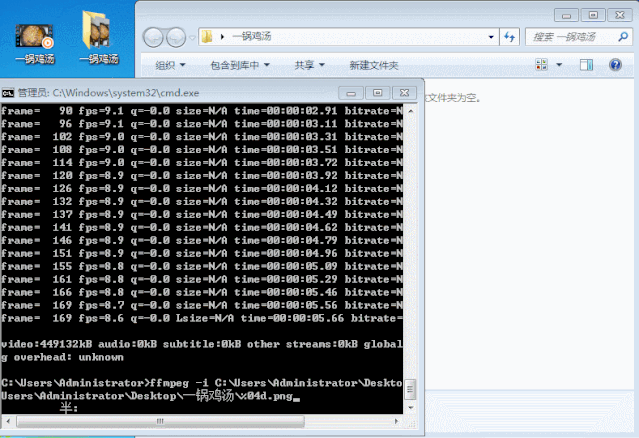2. ffmpeg一行代码实现视频转换为gif动画由于TX对视频的限制，上传的视频必须要经过审核。对于一些时间比较短的视频我们可以将其保存为GIF文件，更方便传播。视频文件保存成GIF格式，是把每一帧保存到GIF的动画帧中，使用该命令时必须将像素格式设置为rgb24。视频转换为gif动画代码如下：ffmpeg -i test.avi -pix_fmt rgb24promotion.gif假如我们有一个需求是将上面那个鸡汤视频命名为“新鲜鸡汤”保存为GIF动画，测试代码如下所示：ffmpeg -i C:\Users\Administrator\Desktop\一锅鸡汤.mp4-pix_fmt rgb24 C:\Users\Administrator\Desktop\一锅鸡汤\新鲜鸡汤.gif生成过程如下所示，只需要短短几秒即可以实现功能，代码中视频和图片格式可以随意进行更换！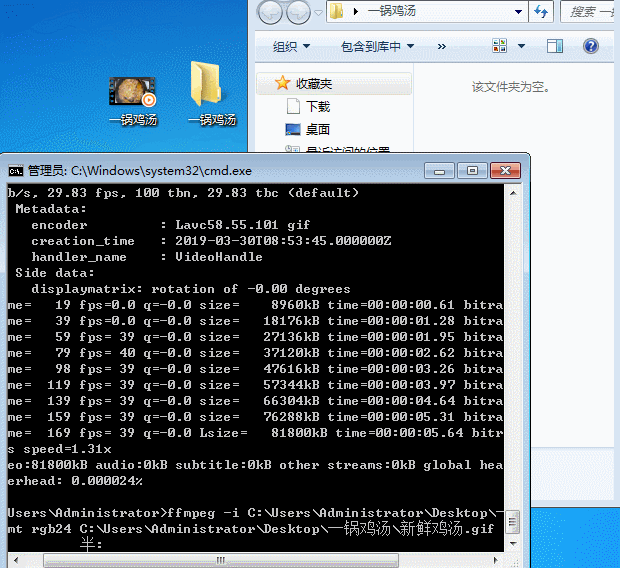3. ffmpeg一行代码实现图片格式转换如果你有一个jpg格式的图片想转换成png格式等类似的格式转换需求，那么可以参考下文方法。Ffmpeg支持除EXR、LJPEG、PIC和PTX文件类型之外的几乎所有图像类型，这极大的方便了我们处理不同格式文件的工作。格式转换的代码如下：ffmpeg -i image.type1 image.type2假设我们现在有个将png转换成jpg格式的需求，即将上文中0001.png格式转换为jpg并以名称“image”保存在相同文件夹内，测试代码如下：ffmpeg -i C:\Users\Administrator\Desktop\0001.pngC:\Users\Administrator\Desktop\一锅鸡汤\image.jpg实现过程如下所示，新生成的image.jpg在右上方文件夹内：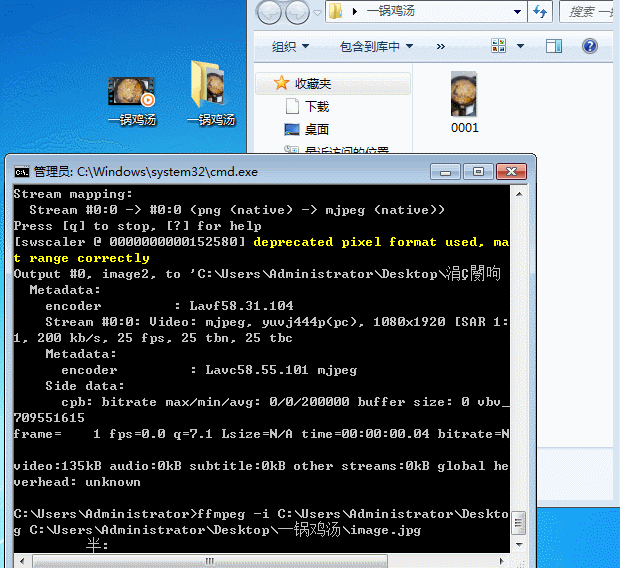此外还有一些音频、视频、图片等格式转换、裁剪编辑等相关问题，请自行了解吧，总之ffmpeg功能多多，操作也非常简单！有了这些图片，我们后续可以做很多好玩的事情，比如针对每一帧图片进行处理，二值化、图像识别、做各种各样的炫酷视频等。如果你想处理的工作内容比较少也比较单一，那么了解上面那么多就够了，如果你想再简单一些或者想批量处理视频、音频、图片等，那么Python会是你很好的工具。假如我们现在有个需求：把上文1000张png格式的图片全部转换为jpg格式，这时如果我们一张张的进行转换，工作量非常大，几乎把自己坑死！！！这时如果借助Python将非常简单，喝杯咖啡的功夫，工作就自动完成了，so easy！4. ffmpeg+Python实现图片格式批量转换针对上面的需求，实现起来非常简单，只需要15行代码！首先获取png图片的存放路径，然后读取路径中所有的图片；再进行格式转换；最后保存到同一个文件夹即可，具体执行代码如下：import subprocessimport os  def png2jpg(png_path, jpg_path=None):   filenames = os.listdir(png_path)   print(filenames)  # 显示文件夹内所有文件名称   for i in range(len(filenames)):       if "png" == filenames[i].split(".")[-1]:           png_files = os.path.join(png_path + "\\" + filenames[i])           jpg_path = os.path.join(png_path + "\\" + filenames[i].rsplit('.',1) + ".jpg")           error = subprocess.call([r"C:\ffmpeg\bin\ffmpeg","-i", png_files, jpg_path])           if error:                print("Done！%s 转换成功！" %filenames[i])       else:           print("%s 不是一个有效的png格式文件！" % filenames[i])  if __name__ == "__main__":   png_path = r"C:\Pythonwork\image2image\一锅鸡汤"  # 定义wav文件所在文件夹   png2jpg(png_path)为了能更直观的看到图片格式转换的过程，我制作了一个GIF图片。为了减小图片的尺寸，此处选择10张照片进行示意，整个转换过程大概不到1秒钟，是不是非常快呀！过程如下所示：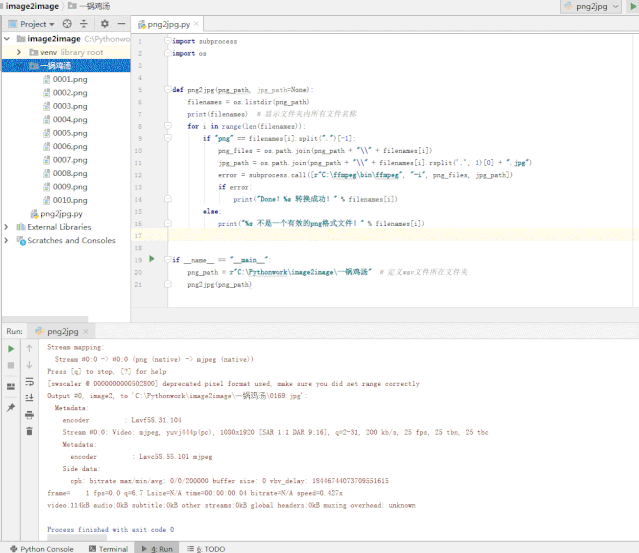附录1 ffmpeg支持的图片格式表格中列出了ffmpeg支持的图像格式及其特征后缀。除LJPEG(无损JPEG)以外的所有这些文件类型都可以解码，除EXR，PIC和PTX之外都可以编码。ffmpeg支持的图片格式：扩展名编码解码描述.Y.U.V√√每个组件的一行文件BMP√√微软BMP图像DPX√√数码照片交换EXRX√OpenEXRGIF√√动画gif是未压缩的JPG√√不支持渐进式JPEGJP2√√JPEG 2000JLS√√JPEG-LSLJPGXX无损的JPEGPAM√√PAM是带有alpha支持的PNM扩展PBM√√便携式位图图像PCX√√PC画笔PGM√√便携式GrayMap形象PGMYUV√√PGM与U和V的分量在YUV 4:2 0PICX√Pictor/PC PaintPNG√√便携式网络图形PPM√√便携式PixelMap形象PTXX√V.Flash  PTX格式SGI√√SGI RGB图像格式RAS√√Sun  Rasterfile图像格式TIFF√√YUV, JPEG和一些扩展还没有被支持TGA√√Truevision  Targa 图像格式XBM√√X位图图像格式XFace√√XFace图像格式XWD√√X窗口转储图像格式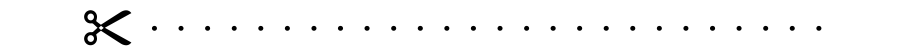python时域信号统计分析Python加速度信号转速度位移小程序Python批量转换音乐视频格式Python编写加速度-振幅-频率转换程序Python程序代码打包成exe独立可执行程序

展开全文• 作者：Tarun Guptadeephub翻译组：孟翔杰​ 在这篇文章中，我们将看一个使用NumPy作为数据处理库的Python3编写的程序，来了解如何实现使用梯度下降法的(批量)线性回归。​ 我将逐步解释代码的工作原理和代码的每个...
• 手写批量线性回归算法：在Python3中梯度下降方法实现模型训练 作者：Tarun Guptadeephub翻译组：孟翔杰 ​ 在这篇文章中，我们将看一个使用NumPy作为数据处理库的Python3编写的程序，来了解如何实现使用梯度下降法...
• 2. 理解数据2.1 导入数据从Kaggle 中下载2.2 导入数据import pandas as pdimport numpy as npimport matplotlib.pyplot as pltimport seaborn as sns导入训练数据与测试数据批量进行数据清洗。#训练数据...
• ## 使用 python 实现 Logistic 回归

千次阅读 多人点赞 2021-04-04 17:55:46
使用 python 实现 Logistic 回归原理回顾预测函数代价函数参数更新代码分析算法的实现算法的使用算法的对比全部代码 这节课我们将使用 numpy 实现逻辑回归算法，然后利用我们自己写的算法在乳腺癌数据集上进行癌症...
• 在这篇文章中，我们将看一个使用NumPy作为数据处理库的Python3编写的程序，来了解如何实现使用梯度下降法的(批量)线性回归。​ 我将逐步解释代码的工作原理和代码的每个部分的工作原理。​ 我们将使用此公式计算梯度...
• 作者：Tarun Guptadeephub翻译组：孟翔杰​ 在这篇文章中，我们将看一个使用NumPy作为数据处理库的Python3编写的程序，来了解如何实现使用梯度下降法的(批量)线性回归。​ 我将逐步解释代码的工作原理和代码的每个...
• ​ 在这篇文章中，我们将看一个使用NumPy作为数据处理库的Python3编写的程序，来了解如何实现使用梯度下降法的（批量）线性回归。 ​ 我将逐步解释代码的工作原理和代码的每个部分的工作原理。 ​ 我们将使用此公式...深度学习 机器学习 算法 人工智能
• 数据散点图和解直线 总结 以上就是本文关于Python编程实现线性回归批量梯度下降法代码实例的全部内容，希望对大家有所帮助。感兴趣的朋友可以继续参阅本站： 如有不足之处，欢迎留言指出。感谢朋友们对本站的支持...
• 数据散点图和解直线 总结 以上就是本文关于Python编程实现线性回归批量梯度下降法代码实例的全部内容，希望对大家有所帮助。感兴趣的朋友可以继续参阅本站： 如有不足之处，欢迎留言指出。感谢朋友们对本站的支持...
• 通过学习斯坦福公开课的线性规划和梯度下降，参考他人代码自己做了测试，写了个类以后有时间再去扩展，代码注释以后再加，作业好多： ...4.python批量梯度下降参考代码：http://www.91r.net/ask/17784587.html
• 在本文中，我们将在Python中构建一个线性回归模型，并应用梯度下降的优化技术来降低成本函数。以下是我们将要讨论的主题：线性回归：模型的解释和概念;梯度下降：关于GD及其变化的解释;代码实现：通过编写Python代码...
• 本文在我的前一篇博客（【深度学习】线性回归（一）原理及python从0开始实现）的基础上，介绍一下深度学习中的小批量随机梯度下降方法（mini-batch stochastic gradient descent），将其应用在线性回归中。...MXNet
• 在开源的基础上实现的逻辑回归，纯python实现，采用的是批量梯度下降，用户可以自己换其他的梯度下降方式
• 关于数据的批量回归插补缺失值 缺失值是数据分析工作中常见的数据问题，我们通常通过中位数、众数、平均数等进行缺失值插补，对于需要高精度插补的水文气象等数据，我们经常使用回归的方式插补，但是，利用excel回归...sklearn
• 前言在上一篇文章《机器学习之Logistic回归(非线性回归)》中我们对Logistic回归算法做了简单的描述，同时也说到了梯度下降算法，我们之前说过，Logistic回归是解决分类问题的模型，同线性回归形似但从根本上来说还是...
• 前言概述上一篇文章对逻辑回归的原理和基本思想做了一些简要介绍，并通过引入Sigmoid函数和梯度公式成功推导出了梯度上升和梯度下降公式，上文分类实例是依据全批量提升上升法，而本文会介绍全批量梯度上升的一种...
• 在机器学习中，有时候需要对原始的模型构造损失函数，然后通过优划算法对损失函数进行优划，从而找到最优的参数使损失函数达到...梯度下降的方式分为三种：批量梯度下降(Batch Gradient Descent)，随机梯度下降(...
• 说明：本文使用的工具为Python3+Jupyter Notebook。 利用批量梯度下降 先导入要用到的各种包： %matplotlib notebook import numpy as np import pandas as pd import matplotlib.pyplot as plt 构造并查看数据...
• 'cubic', 'previous', 'next' 批量处理数据batch_processing() 函数原型 batch_processing(fn, file_path='table1.xls') fn为函数列表，可同时对数据进行多次操作并写入excel，如果只有一个函数，可直接以函数作为...
• else: print('k:%f b:%f err:%f'%(k,b,E)) E1 = E E = 0 #将E重置 print('Over,k=%f,b=%f'%(k,b)) print('%dtimes'%(time)) 运行结果： 如此，一元线性回归批量梯度下降法(BGD)便完成了，除了BGD，还有随机梯度...机器学习 算法...

# python批量回归python 订阅